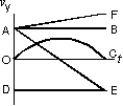Motion in Two and Three Dimensions

# Fundamentals of Physics Study Set 2

Physics & Astronomy

## Quiz 4 :Motion in Two and Three DimensionsStudy FlashcardsLooking for Introductory Physics Homework Help?## Quiz 4 :Motion in Two and Three Dimensions

Showing 1 - 20 of 53Which of the following is a scalar quantity?
Free
Multiple Choice

AVelocity is defined as:
Free
Multiple Choice

AWhich of the following is a vector quantity?
Free
Multiple Choice

ESally starts from home, walks 300 m east and then 500 m south, and arrives at her school.How far, in a straight line, is Sally's school from her home?
Multiple ChoiceFrom your window you can see a high-rise building several blocks away.You check the map and see that the building is 560 m away from you in a straight line, in a direction 30° south of east.However, the roads are all east-west and north-south.If you want to walk from your house to the building, how far in each direction do you have to walk?
Multiple ChoiceA particle goes from x = -2 m, y = 3 m, z = 1 m to x = 3 m, y = -1 m, z = 4 m.Its displacement is:
Multiple ChoiceA jet plane in straight horizontal flight passes over your head.When it is directly above you, the sound seems to come from a point behind the plane in a direction 30 from the vertical.The speed of the plane is:
Multiple ChoiceWhat is the difference between speed and velocity?
Multiple ChoiceAn object moves from x = -2.1 m, y = 3.7 m, z = 1.4 m to x = 3.3 m, y = -1.1 m, z = 4.2 m in a time of 5.3 s.What is its average velocity?
Multiple ChoiceAn object moves from x = -2.1 m, y = 3.7 m to x = 3.3 m, y = -1.1 m in a time of 5.3 s.What is its average velocity?
Multiple ChoiceA plane traveling north at 200 m/s turns and then travels south at 200 m/s.The change in its velocity is:
Multiple ChoiceAcceleration is defined as:
Multiple ChoiceWhich of the following is NOT an example of accelerated motion?
Multiple ChoiceAn object has a velocity of (5.4 m/s)− (4.8 m/s).Over a period of 1.3 s, its velocity changes to (1.7 m/s)+ (5.9 m/s).What is its acceleration?
Multiple ChoiceThe velocity of an object as a function of time is given by v = (12.5t − 7.2t2)+ (4.3t3).What is its acceleration as a function of time?
Multiple ChoiceAn object is at rest at x = 0 m, y = 0 m.It accelerates at a rate of (−3.2 m/s2)+ (8.6 m/s2).After accelerating for 4.7 seconds its coordinates are:
Multiple ChoiceTwo bodies are falling with negligible air resistance, side by side, above a horizontal plane.If one of the bodies is given an additional horizontal acceleration during its descent, it:
Multiple ChoiceThe velocity of a projectile equals its initial velocity added to:
Multiple ChoiceWhich of the curves on the graph below best represents the vertical component vy versus t for a projectile fired at an angle of 45 above the horizontal?